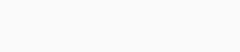# Unit Cube Methodology

31 August 2020, 06:26 am

# Unit Cube Methodology

Early on in my engineering career, when I was doing a lot of simulations and numerical modelling, I got introduced to this concept of the ‘Unit Cube Approach’. I found this particularly useful whilst trying to understand how the system/model in question will behave under the applied restraints or boundary conditions. The objective of this approach was to arrive at an approximation of how the system would behave without consuming too much time. After all, time is one of the most valuable resources we have in our hands and staying in control of it, helps us stay in control of the project.

So, what is a unit cube? The idea is to reduce an analysis problem to its simplest form to get a basic understanding of the system’s behaviour whilst subjecting it to actual loading conditions. From a simulation and numerical modelling perspective, a unit cube when used with certain underlying approximate symmetries to the real problem often set us on the right track very early on. It has the potential to validate two critical things for us:

1. The direction and pattern of deformation/displacement of the system.
2. Reaction forces (mass*applied gravity).

Imagine you are tasked with analysing the behaviour of a complicated piece of kit subjected to inertial loads, forces, pressures etc. Modelling the real thing often takes time, meshing, setting up and running, before you get to look at the results. Given the tight timelines of projects (we engineers know that, right!) the margin for errors and reworks is seldom there. We’re also left with the question of ‘whether it is behaving how it should’.

Let’s recreate the problem using a unit cube. It’s a cube. So, it’s homogenous, i.e. it is the same when looked through the three dimensions of space, which we will call x, y and z. Secondly, because it is a uniform shape, it makes life easier from a meshing point of view. It enables us to create nice chunky elements with perfect aspect ratio, skewness etc (I won’t bore you with mesh quality here). Thirdly, because it has six flat faces, applying of boundary conditions/restraints and loads is also made simple. Now, after meshing and applying the loads and restraints, we run the model and after a few minutes (or seconds), we have a solution.

We then observe the behaviour of the cube under the applied conditions. Whilst quantities like stresses, failure, strength etc. depend on component size, shape, cross-section, material and other things, getting an idea of the cube’s deformation and the net force reactions in the three directions (x, y and z) should put us on the right path, when we look at the actual model.